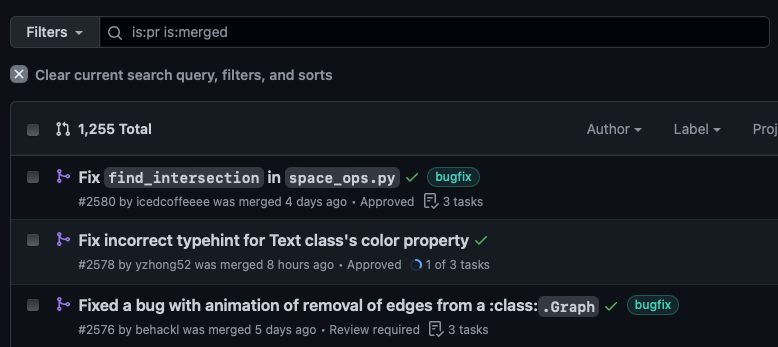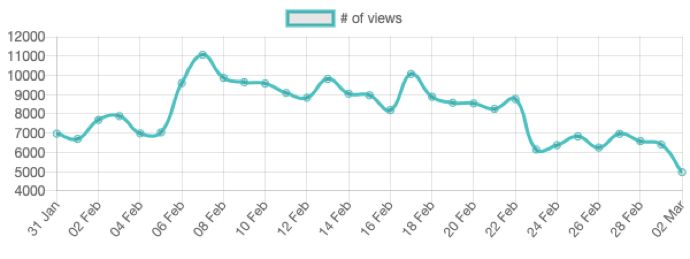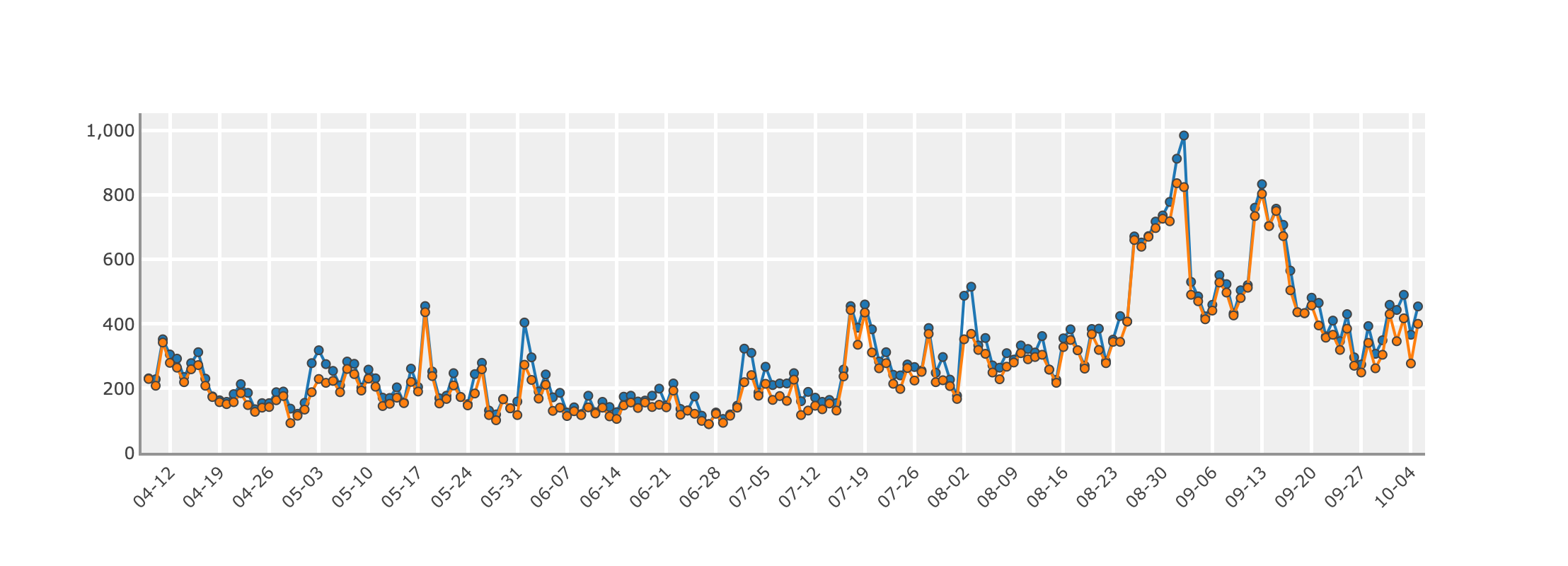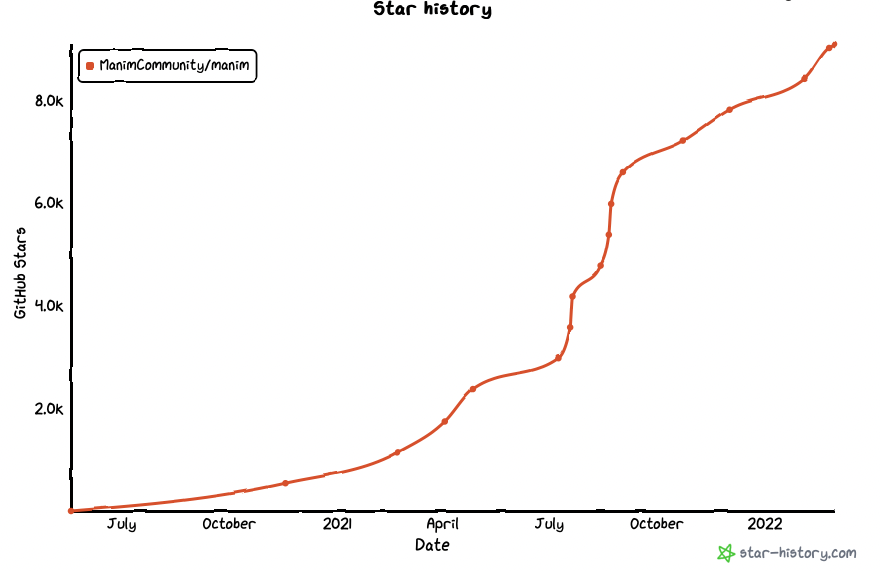Hands-On Manim Workshop
Benjamin Hackl • 3th March, 2022

## Mathematical Animations with Manim

### A brief history of Manim and its versions

• 3b1b/manim: in development since 2015, until 2018 as single-person[Grant Sanderson] project
• May 2020: Formation of a small community, #1 was merged!
• Since then: ~1250 PRs by over 75 contributors! 🤯### Some more statistics

• Daily documentation page visits: around 8000
• Over 9000 ⭐️ on GitHub### Manim and Jupyter notebooks

• Use arrow keys / mouse to navigate through cells in a notebook, press ▶️ or Shift + Enter to execute cells.
• First step: import Manim. With config some options can be setup, e.g., output video width or log verbosity:
from manim import *

config.media_width = "80%"
config.verbosity = "WARNING"

### A first animation!

%%manim -qm CircleToSquare

class CircleToSquare(Scene):
def construct(self):
blue_circle = Circle(color=BLUE, fill_opacity=0.5)
green_square = Square(color=GREEN, fill_opacity=0.8)
self.play(Create(blue_circle))
self.wait()

self.play(Transform(blue_circle, green_square))
self.wait()
• Shapes: Circle, Square
• Animations: Create, Transform
• More in reference manual!

### Positioning Mobjects

• Manim coordinates: 3D coordinate system, by default visible XY plane: $[-7.11, 7.11]\times [-4, 4]$, origin in center of screen.
%%manim -qm HelloCircle

class HelloCircle(Scene):
def construct(self):
circle = Circle()
blue_circle = circle.set_color(BLUE).set_opacity(0.5)

label = Text("A wild circle appears!")
label.next_to(blue_circle, DOWN, buff=0.5)

self.play(Create(blue_circle), Write(label))
self.wait()
• Methods: next_to, move_to, shift
• Constants: UP, DOWN, RIGHT, LEFT

### The .animate syntax – method animations

• Methods like rotate, scale, move_to modify mobjects.
• These changes can be animated in by using .animate!
%%manim -qm CircleAnnouncement

class CircleAnnouncement(Scene):
def construct(self):
blue_circle = Circle(color=BLUE, fill_opacity=0.5)
announcement = Text("Let us draw a circle.")

self.play(Write(announcement))
self.wait()

self.play(announcement.animate.next_to(blue_circle, UP, buff=0.5))
self.play(Create(blue_circle))

### The .animate syntax – method animations

• Multiple methods can be applied at once by chaining them.
%%manim -qm AnimateSyntax

class AnimateSyntax(Scene):
def construct(self):
triangle = Triangle(color=RED, fill_opacity=1)
self.play(DrawBorderThenFill(triangle))
self.play(triangle.animate.shift(LEFT))
self.play(triangle.animate.shift(RIGHT).scale(2))
self.play(triangle.animate.rotate(PI/3))

### The .animate syntax – method animations

• Caution: .animate works by directly interpolating between initial and target mobject, this can lead to unintended effects.
%%manim -qm DifferentRotations

class DifferentRotations(Scene):
def construct(self):
left_square = Square(color=BLUE, fill_opacity=0.7).shift(2*LEFT)
right_square = Square(color=GREEN, fill_opacity=0.7).shift(2*RIGHT)
self.play(left_square.animate.rotate(PI),
Rotate(right_square, angle=PI), run_time=2)
self.wait()

### Manim and LaTeX

• Full support!
• Note: "\" in Python strings need to be escaped (\\), or a raw string (r"...") needs to be used.
%%manim -qm CauchyIntegralFormula

class CauchyIntegralFormula(Scene):
def construct(self):
formula = MathTex(
r"[z^n]f(z) = \frac{1}{2\pi i}\oint_{\gamma} \frac{f(z)}{z^{n+1}}~dz"
)
self.play(Write(formula), run_time=3)
self.wait()

### Manim and LaTeX

• There is syntactic sugar for easier transformations: strings get split according to double braces {{ ... }}.
%%manim -qm TransformEquation

class TransformEquation(Scene):
def construct(self):
eq1 = MathTex("42 {{ a^2 }} + {{ b^2 }} = {{ c^2 }}")
eq2 = MathTex("42 {{ a^2 }} = {{ c^2 }} - {{ b^2 }}")
eq3 = MathTex(r"a^2 = \frac{c^2 - b^2}{42}")
self.wait()
self.play(TransformMatchingTex(eq1, eq2))
self.wait()
self.play(TransformMatchingShapes(eq2, eq3))
self.wait()
• Specialized transforms: TransformMatchingTex, TransformMatchingShapes

### Plotting

%%manim -qm PlotDemo

import numpy as np

class PlotDemo(Scene):
def construct(self):
ax = Axes(x_range=[-1, 5, 1], y_range=[-2, 2, 1])
f = lambda x: np.sin(x*PI) * x/2
plot = ax.plot(f, color=GREEN)
area = ax.get_area(plot, x_range=[1, 3])
self.wait()
self.play(Create(plot))
self.play(DrawBorderThenFill(area))
self.wait()

### Graphs

%%manim -v WARNING -qm ErdosRenyiGraph

import networkx as nx

nxgraph = nx.erdos_renyi_graph(14, 0.5)

class ErdosRenyiGraph(Scene):
def construct(self):
G = Graph.from_networkx(nxgraph, layout="spring", layout_scale=3.5)
self.play(Create(G))
self.play(*[G[v].animate.move_to(5*RIGHT*np.cos(ind/7 * PI) +
3*UP*np.sin(ind/7 * PI))
for ind, v in enumerate(G.vertices)])
self.play(Uncreate(G))